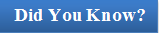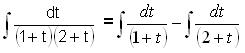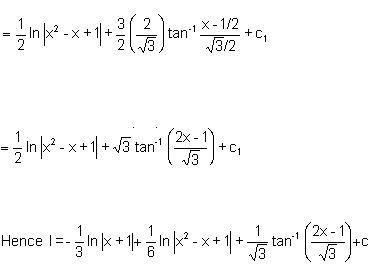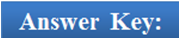×#### Thank you for registering.

One of our academic counsellors will contact you within 1 working day.

Click to Chat

1800-1023-196

+91-120-4616500

CART 0

• 0

MY CART (5)

Use Coupon: CART20 and get 20% off on all online Study Material

ITEM
DETAILS
MRP
DISCOUNT
FINAL PRICE
Total Price: Rs.

There are no items in this cart.
Continue Shopping```Integration By Partial FractionsA function of the form P(x)/Q(x), where P(x) and Q(x) are polynomials, is called a rational algebraic function or a rational function of x. Consider the rational function (x+7)/(2x – 3)(3x + 4) = 1/2x–3 – 1/3x+4

The two fractions on the RHS are called partial fractions. To integrate the rational function on the LHS, it is sufficient to integrate the two fractions on the RHS, which are easily integrable. This is known as the  method of partial fractions. In case the degree of P(x) (numerator) is not less than that of Q(x) (denominator), we carry out the division of P(x) by Q(x) and reduce the degree of the numerator.

In order to write P(x)/Q(x) in partial fractions, first of all we write

Q(x) = (x – a)k ... (x2 + αx + β)r ... where binomials are different, and then setwhere A1, A2, ..., Ak, M1, M2, ......, Mr, N1, N2, ...... ,Nr are real constants to be determined. These are determined by reducing both sides of the above identity to integral form and equating the coefficients of equal powers of x, which gives a system of linear equations in the coefficient. (This method is called the method of comparison of coefficients). The constants can also be obtained by substituting suitably chosen numerical values of x on both the sides of the identity.

Note: Before proceeding to write a rational function as a sum of partial fractions, one must try to figure out whether it is a proper rational fraction or is rewritten as one.A rational function P(x)/Q(x) is proper if the degree of polynomial Q(x) is greater than the degree of the polynomial P(x). In case the degree of P(x) greater than or equal to the degree of Q(x), we first write P(x)/Q(x) = h(x) + P(x)/Q(x), where h(x) is a polynomial and p(x) is a polynomial of degree less than the degree of polynomial Q(x).

Similarly, the function P(x)/Q(x) is said to be an improper rational function if deg P(x) ≥ deg q(x).

We now discuss the various cases for solving questions by partial fractions:

Suppose we have a function of the form f(x)/g(x), then we have the following cases:

1. If the denominator is expressible as the product of non-repeating linear factors i.e.

Let g(x) = (x – a1)(x – a2)(x – a3)........... (x – an).

In such a case we assume that

f(x)/g(x) = C1/(x-a1) + C2/(x-a2) + ….. Cn/(x-an)

, where C1, C2, ...Cn are constants to be determined by equating the numerators of both the sides and then substituting the values of x as a1, a2, …, an.

2. If the denominator is expressible as the product of non-repeating linear factors i.e.

Let g(x) = (x-a)k(x-a1)(x-a2)(x-a3)........... (x-ar).

In such a case we assume that

f(x)/g(x) = C1/(x-a) + C2/(x-a)2 + C3/(x-a)2 + ….. + Ck/(x-a)k + D1(x-a1) + D2(x-a1) + …..... + Dr/(x-ar).

where C1, C2, ...Ck and D1, D2, ...Dr are constants to be determined.

Note: Some of these constants are determined by equating the numerators of both the sides as done in previous case. Remaining constants can be determined by equating the coefficients of like powers on both the sides.

Watch this Video for more reference

3. When some of the factors of denominator g(x) are quadratic but non-repeating.

Supose we have quadratic factors of the type ax2 + bx + c, then the partial fractions should be assumed to be of the type (Ax + B)/(ax2 + bx + c), where A and B are constants which can be computed by comparing the coeffcients of like powers of x in the numerators of both the sides. In fact, partial fractions in this case should better be assumed as

A(2ax + b)/(ax2 + bx + c) + B/(ax2 + bx + c).

4. When some of the factors of the denominator g(x) are quadratic and repeating.

Corresponding to every quadratic repeating factor of the type (ax2 + bx + c)l, we assume 2l partial fractions of the type

[A0(2ax + b)/(ax2 + bx + c) + A1/(ax2 + bx + c)] + [A1(2ax + b)/(ax2 + bx + c)2 + A2/(ax2 + bx + c)2] + …................ + [A2l -1(2ax + b)/(ax2 + bx + c)l + A2l/(ax2 + bx + c)l].It is always better to chooose the most appropriate partial fractionHere, A, B and C are real numbers to be suitably determined.

We now discuss certain integrations using partial fractions:

Example 1:

Evaluate ∫ cos x dx/(1 + sin x)(2 + sin x).

Solution:

Put sinx = t ⇒ cosx dx = dt= log (1 + t) – log (2 + t) + c

= log (1 + sin x)/(2 + sin x) + c

______________________________________________________________________________

Example 2:

Evaluate ∫1/sin x (2 + cos x – 2 sin x) dx.

Solution:

Put tan = t ⇒ sec2 x/2 . 1/2 dx = dt

⇒ dx = 2/sec2 x/2 dt

⇒ dx = 2/(1 + t2) dt, then we haveExpands into simple fractions

1 + t2/t(t–3) = A/t + B/(t–3) + C/(t–1)

After solving the coefficients, A = 1/3; B = 5/3; C = –1

Hence I = 1/3 ∫ dt/t + 5/3 ∫ dt/(t–3) – ∫ dt/(t-1)

= 1/3 In |t| + 5/3 |t–3| – In |t–1| + c

= 1/3 In | tan x/2 | + 5/3| tan x/2 –3 | – ln | tan x/2 – 1 | + c.

______________________________________________________________________________

Example 3:

Evaluate ∫ x dx/(x3 + 1).

Solution:

Since x3 + 1 = (x + 1) (x2 - x + 1) (the second factor is not a product of linear factors), hence the partial fractions of the given integer will have the form.

x/(x3 + 1) = A/(x + 1) + Bx + D/(x2 - x + 1)

Hence, x = A(x2 - x + 1) + (Bx + D)(x + 1) = (A + B)x2 + (-A + B + D)x + (A + D).

Equating the coefficients of equal powers of x, we get A = -1/3, B = 1/3, D = 1/3.

Thus l = – 1/3 ∫dx/(x +1) + 1/3 ∫(x+1)/(x2 - x + 1) dx = – 1/3 |x + 1| + 1/3 l1.

To calculate the integral l1 = ∫(x + 1)/(x2 – x + 1)dx.

Express (x +1) = l (d.c. of x2 - x + 1) + m

⇒ (x + 1) = l(2x - 1) + m = 2xl - l + m

Comparing the coefficients of like powers of x, we get l =1/2 and m = 3/2

⇒ l1 = 1/2 ∫(2x–1)/(x2 - x + 1) dx + 3/2 ∫dx/(x2 - x + 1).Q1. The suitable partial fraction for the rational function of the form (px + q)/(x-a)(x-b) is

(a) C/(x-a) + D/(x-b)

(b) C/(x-a)(x-b)

(c) C/(x-a)2 + D/(x-b)2

(d) none of these

Q2. In an improper rational function,

(a) degree of numerator = degree of denominator

(b) degree of numerator ≥ degree of denominator

(c) degree of numerator ≤ degree of denominator

(d) degree of numerator > degree of denominator

Q3. When the denominator is a combination of linear and quadratic factors, the suitable partial fractions are

Q3. When some of the factors of the denominator are quadratic and repeating such as (px2 + qx + r)t, then

(a) we assume ‘t’ partial fractions

(b) we assume ‘t/2’ partial fractions

(c we may assume ‘2t’ or ‘t’ partial fractions

(d) we assume ‘2t’ partial fractions

Q4. The partial fractions for the rational function (px2 + qx + r)/(x-c)(x2 + dx + e) are

(a)  A/(x - c) + (Bx2 + C)/(x2 + dx + e)

(b)  A/(x - c)2 + (Bx + C)/(x2 + dx + e)

(c) A/(x - c) + (Bx + C)/(x2 + dx + e)

(d) None of theseQ1.

Q2.

Q3.

Q4.

(a)

(b)

(d)

(c)

Related Resources

For getting an idea of the type of questions asked, refer the previous year papers.

You may wish to refer indefinite integral.

To read more, Buy study materials of Indefinite integral comprising study notes, revision notes, video lectures, previous year solved questions etc. Also browse for more study materials on Mathematics here.

```### Course Features

• 731 Video Lectures
• Revision Notes
• Previous Year Papers
• Mind Map
• Study Planner
• NCERT Solutions
• Discussion Forum
• Test paper with Video Solution